Troitskii V.A.

Russia,St.Petersburg,St.Petersburg State Technical University, e-mail: vat@topol. stu. neva. Ru

OPTIMIZATION APPROACHES TO SOME OBSERVATION PROBLEMS FOR PDE

Two-dimension domain is considered. There are two subdomain in it. The sources ofdisturbation are distributed in one of them and the measurements are fulfilled in other. The intensity of the disturbances must be determined if the results of measurements are known. The variation approaches of the optimization theory were used for constructing of the methods of solution and solution such problems.

Троицкий В. А.

Россия,Санкг-Петербург,Санк-Петербургский Государственный Технический Университет,e-mail: vat@itopol.stu.neva.ru

ОПТИМИЗАЦИОННЫЕ ПОДХОДЫ К НЕКОТОРЫМ ЗАДАЧАМ НАБЛЮДЕНИЯ ДЛЯ УРАВНЕНИЙ В ЧАСТНЫХ ПРОИЗВОДНЫХ

Рассматриваются двумерные области, в которых имеются две подобласти. В одной из них распределены источники возмущающих воздействий, а в другой производятся измерения. Должна быть найдена интенсивность источников возмущения по известным результатам измерений. При создании методов решения и при решении таких задач использованы вариационные подходы теории оптимальных систем.

We have a large class of the problems of observation theory and in particular the problems of measurement data interpretation or the diagnostic problems which may be formulated as optimization problems for PDE. We are limited only the consideration of some simple problems of this class.

We consider two-dimensional domain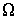with the boundary L and assume that the studying process in this domain is discribed by PDE. We also assume that there are two curves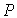and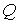in this domain. The sources of disturbances of the process are in. The intencity of these sources is denoted by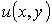. The measurement of characteristic of process is fulfilled in. In the observstion problem we must find the function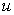if the function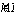is known. [1,2].

It is known that these problem is one of the inverse problem for PDE and that the most part of such problems may be formulated as variational problems [ 3,4 ]. To do it we assume that the function и is unknown and the function v is given. The differential equation of process and the boundary conditions are also given. We construct the functional which gives the estimation of the deviation of the measuring characteristic infor some disturbation from given values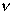. Minimization of this functional must give chance to find the intensity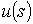of the sources.

By this way our problem is transformed in the problem of the calculus of variations. If we assume that the function и is the control function then we obtain the problem of the theory of optimal processes for two-dimensional PDE [ 6 ].To solve it we may use the first neccessary condition of variations calculus - the rule of multipliers [7,8]. This neccessary condition allows us to get the PDE for main Lagrange's multiplier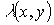, which takes in account initial PDE of our problem, and the conditions, which are neccessary for solving of variational problem. Among these conditions is very important one. It discribes behaviour of the multiplierin.This multiplier may be discontinuity close one-dimension. This fact is the essential pecularity of such variational problems.

The equation and conditions of initial system and conjugate equation and conditions for the Lagrange's multiplier can be used for construction of the numerical methods. These methods have usually bed convergence. This fact is connect with the pecularity of the optimal problems and perhaps with pecularity of inverse problems for PDE . The solution of inverse problems must be found among functions belonging to the compact.Because of these facts we select another way and onstructed numerical procedure based on the gradient method of the first order.

In order to investigate posibilities of this method at first weconsider the simple problem of the heat transfer theory. This problem is a nonstationary one-dimensional heat transfer problem for domain;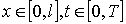, where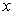is coordinate and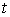is time. In the point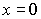we have heat flow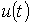and in the point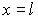we measure the temperature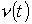. In the interpretation problem we must determineif is given.

The numerical solution of this simple problem by means ofdiscribing gradient method shows very good convergence for different admissible function.This fact is not surprice because solution is automatically found among functions belonging to the compact.That is why this gradient procedure was taken as the method is able to solve more difficult problems.

The second problem is one-dimensional nonstationary heat transfer problem for the same intervals forand.In it the perturbationis in the point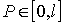and the temperature is measured in the point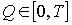.Therefore we have the disconti-nuity of heat flow in the point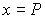in initial problem and the discontinuity of the derivation of multiplier in the point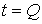in conjugate problem. The gradien procedure was constructed for this problem and it again showed good convergence for different admissible function.

We also consider the heat transfer problem of another type.It is the well knowing problem of heating one-dimesional body for obtaining given distribution of temperature [4,10 ].In this problem we must find functionwhich is equal to heat flow in the pointfor given functionwhich is equal to distribution of the temperature for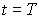.The gradient procedure was also constructed for this problem. We again solve some such problems with different admissible function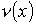.

We also consider following two-dimensional stationary heattransfer problem. The domainis quadrate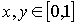. We have the heat sources ofinensityon the line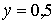and we meassure the temperature on the line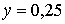. We must findif the temperature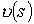on the lineis known. We constructed the computational process based on gradient method for this problem and solved it for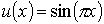. It is taken the function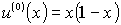for the initial approximation. It is obtained a good convergence of the solution process.

Interpretation

LITERATURE

1.LC.Carthel,R.Glovinski,and J.L.Lions-On exact and approximate boundary controllabilities for the heat equation:A numerical approatch.Joumal of optimization theory and application. 1994, Vol.82,No.3

2.A.Osses,J.P.Puel.Boundary controllability of a stationary Stokes system with linear convection observed on an interior curve. Journal of optimization theory and application. 1998,vol.99.No. 1.

3. Tichonov A.N.,Arsenin V.la. Method for solution of noncorrect problems (in Russian). Nauka,1988

4. Tichonov A.N., Kalner V.D., Glasko V.B. Mathematical models of technology processes.(in Russian). Mashinostroenie.1990

5. Pontriagin L.S.,Boltiansku V.G., Gamkrelidze R-Mischenko M-E-Matematical theory of optimal processes, (in Russian).Nauka.l961

6 Tichonov A.N.,Samarskii AA.The equation of matematical physics.(in Russian). Gostechizdat.1953.

7. Troitskii V.A. Optimal processes in mechanical vibration system.(in Russian). Mashinostroenie.1976.

8. Troitskii V.A.JPetuchov L.V.Shapes optimization of elastic bodies, (in Russian).Nauka. 1982.

9. Marchuk G.LThe methods of calculating mathematics, (in Russian).Nauka. 1990.

10. Egorov A.I. Optimal control of heat and diffusional processes, (in Russian).Nauka. 1978.

 Site of Information Technologies Designed by  inftech@webservis.ru.